# January 2020For this month’s Traders’ Tips, the focus is Jay Leavitt’s article in the October 2019 issue, “An Interplanetary Marriage.” Here, we present the January 2020 Traders’ Tips code with possible implementations in various software.

You can right-click on any chart to open it in a new tab or window and view it at it’s originally supplied size, often much larger than the version printed in the magazine.

The Traders’ Tips section is provided to help the reader implement a selected technique from an article in this issue or another recent issue. The entries here are contributed by software developers or programmers for software that is capable of customization.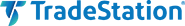In the article in the October 2019 issue titled “An Interplanetary Marriage,” author Jay Leavitt describes the evolution process required in strategy design by introducing his Mars strategy, which grew out of concepts presented in his earlier articles in S&C on his stratosphere, tech emini, and moon rocket ideas. The author uses a linear regression of price data to help derive slope and acceleration information, in turn helping him to identify trends and trend turning points. Leavitt has already provided TradeStation EasyLanguage code for the calculations he uses, and we are showing that code here. This EasyLanguage code can also be downloaded by visiting our TradeStation and EasyLanguage support forum at the link provided below.

```Function: LeavittProjection
// TASC JAN 2020
// Author Jay Leavitt
// function LeavittProjection
inputs:
Price(numericseries),
Length(numericsimple) ;
LeavittProjection  = LinearRegValue(Price, Length, -1) ;

Function: LeavittConv
// TASC JAN 2020
// Author Jay Leavitt
// function LeavittConv
inputs:
Price(numericseries),
Length(numericsimple) ;
vars:
LenConv(0)  ;
LenConv = IntPortion( SquareRoot(Length)) ;
LeavittConv = LinearRegValue(LeavittProjection(Price, Length), LenConv, -1);

Function: LeavittConvSlope
// TASC JAN 2020
// Author Jay Leavitt
// function LeavittConvSlope
inputs:
Price(numericseries),
Length(numericsimple) ;
vars:
LenConv(0)  ;
LenConv = IntPortion( SquareRoot(Length)) ;
LeavittConvSlope = LinearRegSlope(LeavittProjection(Price, Length), LenConv);

Indicator: LeavittConvSlope
// TASC JAN 2020
// Author Jay Leavitt
// indicator LeavittConvSlope
// show plot1 as histogram
inputs:
Price( Close ),
length( 61 ),
UnSure( .2 );
variables:
LCSlope( 0 ) ;

LCSlope = LeavittConvSlope( Price, Length );
Plot1( LCSlope, "LeavittConvSlope" ) ;
Plot2(UnSure,"+UnSure", White);
Plot3(-UnSure,"-UnSure", White);
If LCSlope > LCSlope then
SetPlotColor( 1, green );
If LCSlope < LCSlope then
SetPlotColor( 1, red );
Plot4 ( 0, "ZeroLine" ) ;
```

A sample chart is shown in Figure 1.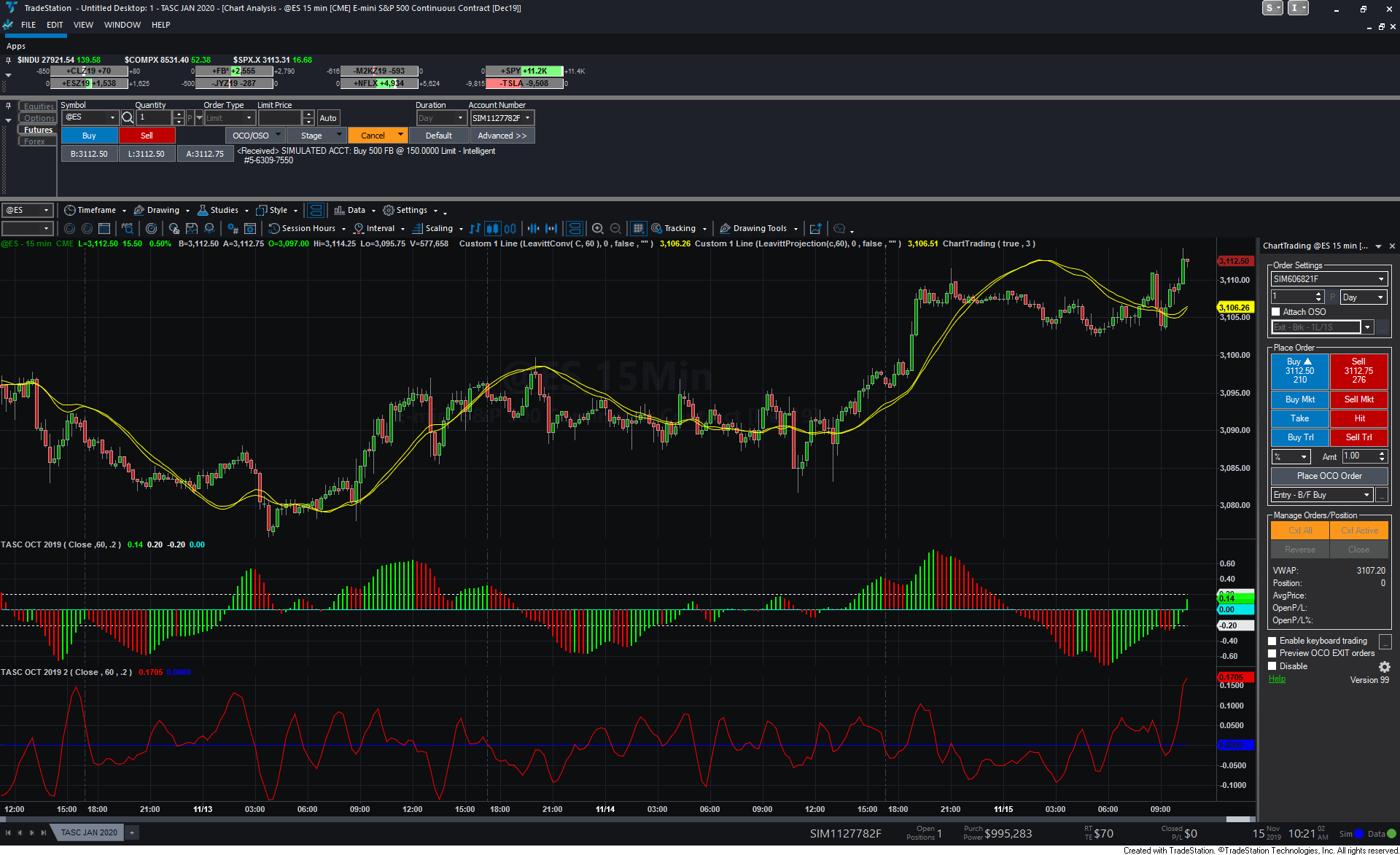FIGURE 1: TRADESTATION. Shown here is a 15-minute TradeStation chart of the emini S&P 500 futures contract with the author’s indicator.

—Doug McCrary

BACK TO LIST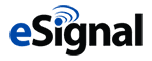### eSIGNAL: JANUARY 2020

For this month’s Traders’ Tip, we’ve provided the study LeavittConvolutionSlope.efs based on the article by Jay A. Leavitt that appeared in the October 2019 issue titled “The Mars Strategy: An Interplanetary Marriage.”

The studies contain formula parameters that may be configured through the edit chart window (right-click on the chart and select “edit chart”). A sample chart is shown in Figure 2.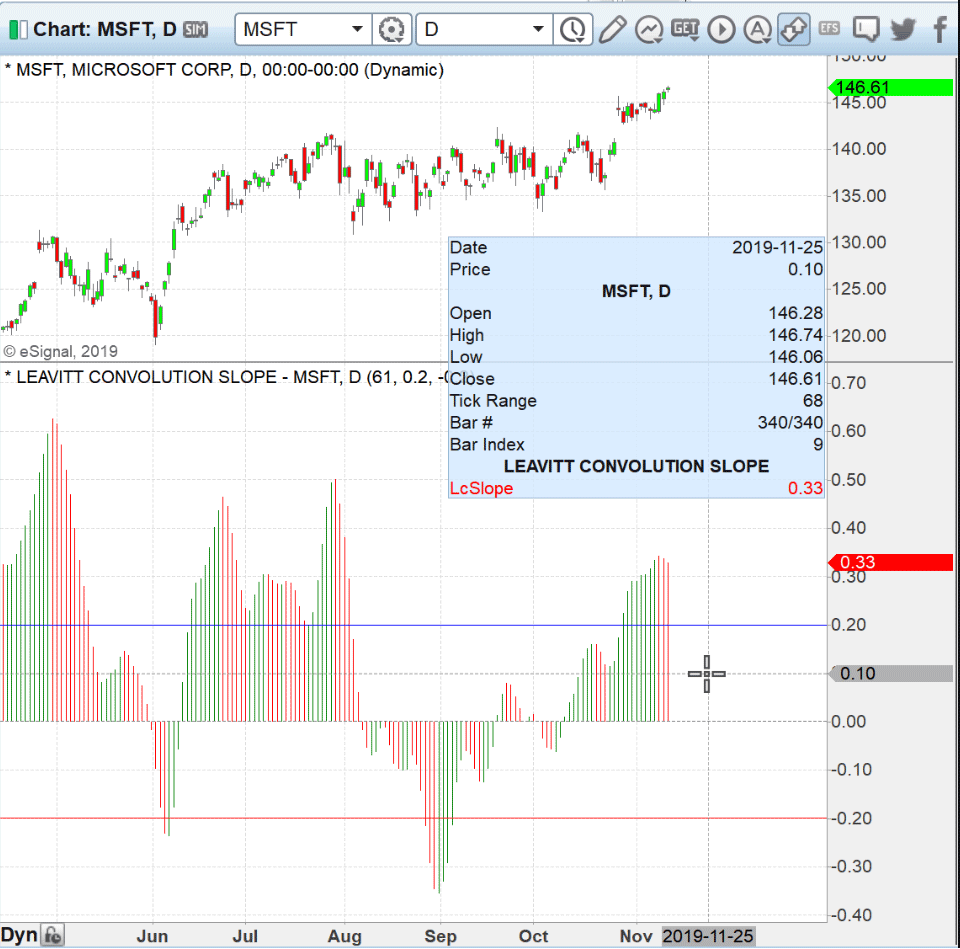FIGURE 2: eSIGNAL. Here is an example of the study plotted on a daily chart of MSFT.

To discuss this study or download a complete copy of the formula code, please visit the EFS Library discussion board forum under the forums link from the support menu at www.esignal.com or visit our EFS KnowledgeBase at www.esignal.com/support/kb/efs/. The eSignal formula script (EFS) is also available for copying & pasting here:

```/**********************************
Provided By:
eSignal is a service mark and/or a registered service mark of Intercontinental Exchange, Inc.
in the United States and/or other countries. This sample eSignal Formula Script (EFS)
is for educational purposes only.
Intercontinental Exchange, Inc. reserves the right to modify and overwrite this EFS file with each new release.

Description:
An Interplanetary Marriage
by Jay A. Leavitt

Version:            1.00  11/07/2019

Formula Parameters:                     Default:
Length                                  61
UnSureUp           						0.2
UnSureLw								-0.2

Notes:
The related article is copyrighted material. If you are not a subscriber

**********************************/
var fpArray = new Array();
var bInit = false;

function preMain() {
setStudyTitle("LEAVITT CONVOLUTION SLOPE");
setCursorLabelName("LcSlope", 0);
setPriceStudy(false);
setDefaultBarFgColor(Color.RGB(0x00,0x94,0xFF), 0);
setPlotType( PLOTTYPE_HISTOGRAM, 0 );

var x=0;
fpArray[x] = new FunctionParameter("Length", FunctionParameter.NUMBER);
with(fpArray[x++]){
setLowerLimit(1);
setDefault(61);
}

fpArray[x] = new FunctionParameter("UnSureUp", FunctionParameter.NUMBER);
with(fpArray[x++]){
setLowerLimit(0);
setDefault(0.2);
}

fpArray[x] = new FunctionParameter("UnSureLw", FunctionParameter.NUMBER);
with(fpArray[x++]){
setUpperLimit(0);
setDefault(-0.2);
}

}

var bVersion = null;
var xClose = null;
var LRV = null;
var LCS = null;
var LenConv = null;

function main(Length, UnSureUp, UnSureLw) {
if (bVersion == null) bVersion = verify();
if (bVersion == false) return;

if ( bInit == false ) {
xClose = close();
LRV = efsInternal("LinearReg", xClose, Length, 1);
LenConv = parseInt( Math.sqrt(Length));
LCS = efsInternal("LinearReg", LRV, LenConv, 0);

bInit = true;
}

if (getCurrentBarCount() <= Length + LenConv ) return;

if (LCS.getValue(0) > LCS.getValue(1)) {
setBarFgColor(Color.red)
}
else if (LCS.getValue(0) < LCS.getValue(1)) {
setBarFgColor(Color.green)
}
else setBarFgColor(Color.blue)

return LCS.getValue(0);
}

function LinearReg(_price, _length, index)
{
var SL = 0;
var SumBars = _length * (_length - 1) * 0.5;
var SumSqrBars = (_length - 1) * _length * (2 * _length - 1) / 6;
var Sum1 = 0;
var SumY = 0;
var i = 0;

for (i = 0; i < _length; i++) {
Sum1 += i * _price.getValue(-i);
SumY += _price.getValue(-i);
}
var Sum2 = SumBars * SumY;
var Num1 = _length * Sum1 - Sum2;
var Num2 = SumBars * SumBars - _length * SumSqrBars;
SL = Num1 / Num2;
var Intercept = (SumY - SL * SumBars) / _length;
LinearRegValue = Intercept + SL * (_length - 1);
if (index == 1) {
return LinearRegValue;
}
else if (index == 0){
return SL;
}
}

function verify(){
var b = false;
if (getBuildNumber() < 779){

drawTextAbsolute(5, 35, "This study requires version 10.6 or later.",
Color.white, Color.blue, Text.RELATIVETOBOTTOM|Text.RELATIVETOLEFT|Text.BOLD|Text.LEFT,
null, 13, "error");
Color.white, Color.blue, Text.RELATIVETOBOTTOM|Text.RELATIVETOLEFT|Text.BOLD|Text.LEFT,
return b;
}
else
b = true;

return b;
}
```

—Eric Lippert
eSignal, an Interactive Data company
800 779-6555, www.eSignal.com

BACK TO LIST### THINKORSWIM: JANUARY 2020

We have put together a study for use in thinkorswim based on the LcSlope that Jay Leavitt mentions in his October 2019 issue article. We built the study referenced by using our proprietary scripting language, thinkScript. To ease the loading process, simply click on http://tos.mx/UoPxtLZ or enter it into setup—open shared item from within thinkorswim, then choose view thinkScript study and name it “leavittconvolutionslope.” This can then be added to your chart from the edit study and strategies menu within thinkorswim.

Figure 3 shows the study on a three-day 30-minute chart of light sweet crude oil futures. Please see Jay Leavitt’s article for more information on how to read this study.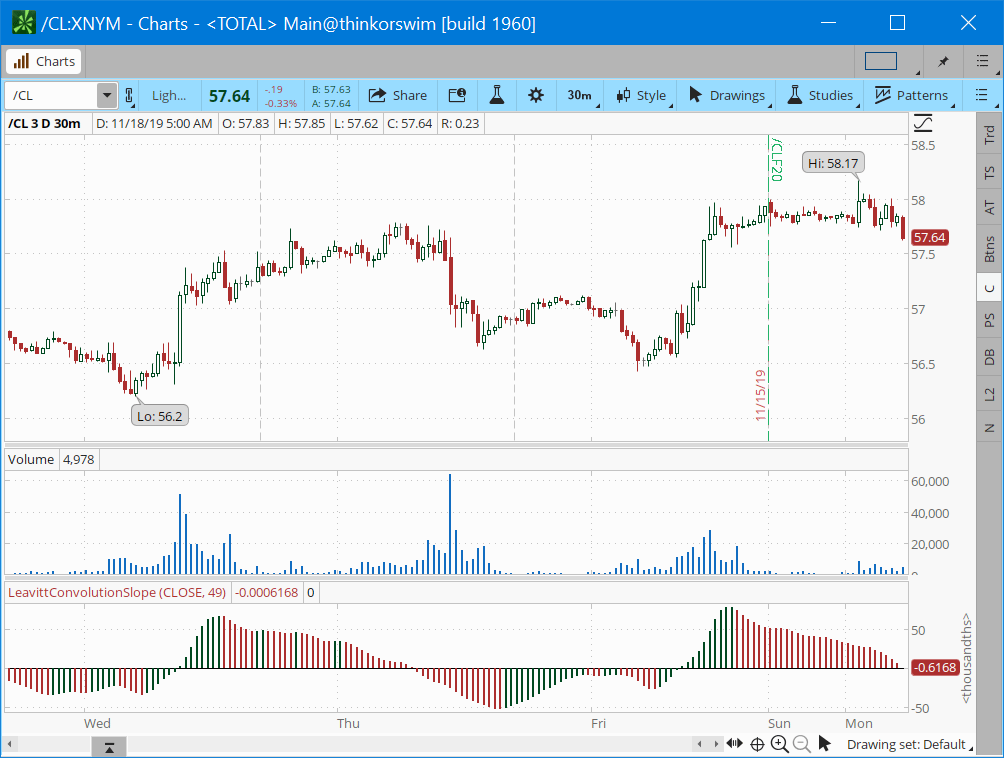FIGURE 3: THINKORSWIM. The study discussed is shown on a 30-minute chart of light sweet crude oil futures.

—thinkorswim
A division of TD Ameritrade, Inc.
www.thinkorswim.com

BACK TO LIST### WEALTH-LAB: JANUARY 2020

This Traders’ Tip is based on Jay Leavitt’s article in the October 2019 issue, “An Interplanetary Marriage.”

The October 2020 article doesn’t provide clear rules with which to build a trading system. Here’s our attempt at creating something:

Entry rules

• Buy next bar at open when the Leavitt convolution slope and the LcAcceleration both get above zero

Exit rules

• Exit at market when the Leavitt convolution slope “reaches its peak” (as defined by rising to its 20-bar high) and then the LcAcceleration changes its sign (that is, goes below zero).

Figure 4 illustrates the system’s mechanics on a sample trade in AXP. In July 2019 the LeavittConvSlope indicator hits a 50-bar highest high, touching the blue dashed line. Shortly afterward, the LcAcceleration indicator turns down and the system is out.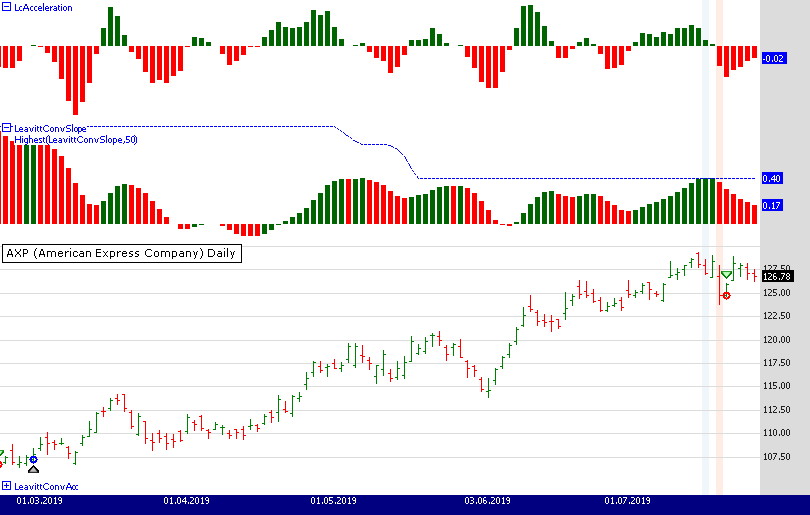FIGURE 4: WEALTH-LAB. This shows the sample trading rules applied to a chart of AXP (American Express). Data provided by Yahoo Finance.

To get the strategy’s C# code, no need to copy/paste; simply download it right from Wealth-Lab’s open strategy dialog.

```WealthScript Code (C#)

using System;
using System.Collections.Generic;
using System.Text;
using System.Drawing;
using WealthLab;
using WealthLab.Indicators;

namespace WealthLab.Strategies
{
public class Leavitt : WealthScript
{
private StrategyParameter paramLength;
private StrategyParameter paramPeak;

public Leavitt()
{
paramLength = CreateParameter("LcP Length", 50, 10, 50, 10);
paramPeak = CreateParameter("LcSlope Peak", 20, 5, 50, 5);
}

protected override void Execute()
{
bool reachedPeak = false, changedSign = false;
int peakBar = -1, signBar = -1;
int peakBars = paramPeak.ValueInt;

int Length = paramLength.ValueInt;
var LeavittProjection = LinearReg.Series(Close, Length);

int LenConv = Convert.ToInt32(Math.Sqrt(Length));
var LeavittConv = LinearReg.Series(LeavittProjection, LenConv);
var LeavittConvSlope = LinearRegSlope.Series(LeavittProjection, LenConv);
LeavittConvSlope.Description = "LeavittConvSlope";

var LcAcceleration = LeavittConvSlope - (LeavittConvSlope>>1);
LcAcceleration.Description = "LcAcceleration";

var LeavittConvAcc = new DataSeries(Bars,"LeavittConvAcc");
LeavittConvAcc.Description = "LeavittConvAcc";

HideVolume();
ChartPane p3 = CreatePane(10,false,false);
ChartPane p2 = CreatePane(30,true,false);
ChartPane p1 = CreatePane(30,true,false);
PlotSeries(p1, LcAcceleration, Color.Blue, LineStyle.Histogram, 5 );
PlotSeries(p2, LeavittConvSlope, Color.Blue, LineStyle.Histogram, 5 );
PlotSeries(p3, LeavittConvAcc, Color.Blue, LineStyle.Histogram, 5 );
PlotSeries( p2, Highest.Series( LeavittConvSlope, peakBars ), Color.Blue, LineStyle.Dashed, 1 );

for(int bar = 1; bar < Bars.Count; bar++)
{
SetSeriesBarColor( bar, LcAcceleration,
LcAcceleration[bar] > 0 ? Color.DarkGreen: Color.Red );

SetSeriesBarColor( bar, LeavittConvSlope,
LeavittConvSlope[bar] > LeavittConvSlope[bar-1] ? Color.DarkGreen: Color.Red );

if( bar > 2 )
{
if (Close[bar - 2] - 2*Close[bar - 1] + Close[bar] > 0)
LeavittConvAcc[bar] = 1;
else
LeavittConvAcc[bar] = -1;
}
}

for(int bar = GetTradingLoopStartBar(1); bar < Bars.Count; bar++)
{
if (IsLastPositionActive)
{
//This exit was taken on the 30-minute chart precisely when
//LcSlope reached its peak and LcAcceleration changed sign.

var hb = HighestBar.Series( LeavittConvSlope, peakBars )[bar];
if( !reachedPeak ) {
if( bar == hb ) {
SetBackgroundColor( bar, Color.FromArgb(50,Color.LightBlue));
reachedPeak = true;
peakBar = bar;
}
}

if( reachedPeak ) {
if( !changedSign ) {
if( LeavittConvSlope[bar] < 0 ) {
SetBackgroundColor( bar, Color.FromArgb(50,Color.LightSalmon));
changedSign = true;
signBar = bar;
}

if( changedSign ) {
if( SellAtMarket( bar+1, LastPosition ) ) {
reachedPeak = false;
changedSign = false;
}
}
}
}
}
else
{
if( LeavittConvSlope[bar] > 0 )
}
}
}
}
}
```

—Gene (Eugene) Geren, Wealth-Lab team
MS123, LLC
www.wealth-lab.com

BACK TO LIST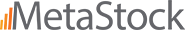### METASTOCK: JANUARY 2020

Several articles by Jay Leavitt have appeared in S&C over the past couple of years. In the December 2019 issue of S&C, Leavitt replied to some letters from readers and included formulas for the calculations used in the strategies discussed in his articles. The MetaStock versions of those formulas are shown here:

```Leavitt convolution
Formula:
x:= Input("Length", 2, 500, 61);
lenconv:= LastValue( Int(Sqrt(x)));
LeavPro:= Ref(LinearReg(C, x), -1);
Ref(LinearReg(LeavPro, lenconv), -1)
Leavitt convolution slope
Formula:
x:= Input("Length", 2, 500, 61);
lenconv:= LastValue( Int(Sqrt(x)));
LeavPro:= Ref(LinearReg(C, x), -1);
LinRegSlope(LeavPro, lenconv)
Leavitt convolution curvature
Formula:
x:= Input("Length", 2, 500, 61);
lenconv:= LastValue( Int(Sqrt(x)));
LeavPro:= Ref(LinearReg(C, x), -1);
LeavConSlope:= LinRegSlope(LeavPro, lenconv);
If(LeavConSlope>Ref(LeavConSlope,-1), LeavConSlope, 0);
If(LeavConSlope<=Ref(LeavConSlope,-1), LeavConSlope, 0);
```

Please note: The formula for the curvature plots two lines, one for increase values and the other for decreasing values. Jay Leavitt suggests setting the increasing values line to green with a histogram line style. Set the decreasing values line to red with a histogram line style. He also adds two lines at values of 0.2 and -0.2 for boundaries within which price direction is unclear.

MetaStock suggests increasing the weight of the histogram lines by one or two levels to make the lines more visible and give the chart a more striking appearance.

—William Golson
MetaStock Technical Support
www.metastock.com

BACK TO LIST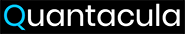### QUANTACULA STUDIO: JANUARY 2020

The indicators described by Jay Leavitt in the December 2019 “Letters To S&C” column include the LeavittProjection, LeavittConv, and LeavittConvSlope. These can be recreated in Quantacula by using the built-in linear regression (LR) and linear regression slope (LRSlope) indicators. The code for Quantacula to construct the indicators is shown below, as well as some example trading rules based on LeavittProjection/Conv crossovers and LeavittConvSlope.

Figure 5 shows the example trading rules implemented on a Quantacula chart.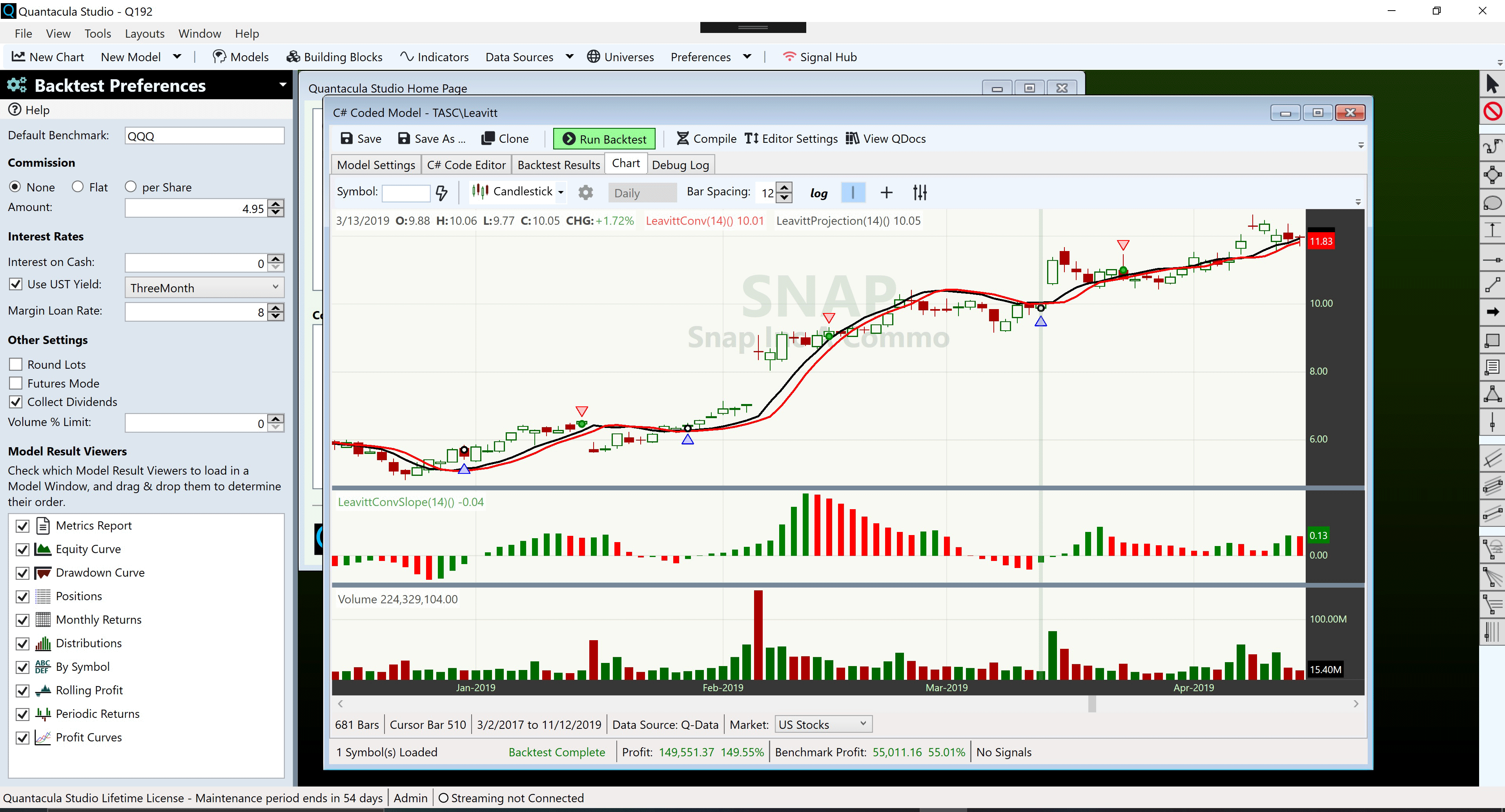FIGURE 5: QUANTACULA STUDIO. This chart demonstrates the Leavitt trading rules on SNAP, illustrating three consecutive winning trades.

```using QuantaculaBacktest;
using System;
using QuantaculaCore;
using QuantaculaIndicators;
using System.Drawing;
using System.Collections.Generic;

namespace Quantacula
{
public class MyModel1 : UserModelBase
{
//variable declarations
private int length = 20;
private TimeSeries LeavittProjection;
private TimeSeries LeavittConv;
private TimeSeries LeavittConvSlope;

//create indicators and other objects here, executed prior to main trading loop
public override void Initialize(BarHistory bars)
{
StartIndex = 21;
LeavittProjection = new LR(bars.Close, length) >> 1;
int p = (int)(Math.Sqrt(length));
LeavittConv = new LR(LeavittProjection, p) >> 1;
LeavittConvSlope = new LRSlope(LeavittProjection, p) >> 1;
PlotTimeSeries(LeavittProjection, "LeavittProjection(14)", "Price", Color.Black);
PlotTimeSeries(LeavittConv, "LeavittConv(14)", "Price", Color.Red);
PlotTimeSeries(LeavittConvSlope, "LeavittConvSlope(14)", "Slope", Color.Green, PlotStyles.Histogram);
}

//execute the strategy rules here, executed once for each bar in backtest history
public override void Execute(BarHistory bars, int idx)
{
//color slope bars green or red
Color c = LeavittConvSlope[idx] > LeavittConvSlope[idx - 1] ? Color.Green : Color.Red;
SetSeriesBarColor(LeavittConvSlope, idx, c);

if (LastPosition == null)
{
//buy rules - look for LeavittProjection crossing over LeavittConv with Slope < 0
if (LeavittProjection.CrossesOver(LeavittConv, idx))
if (LeavittConvSlope[idx] < 0)
}
else
{
//sell rules - exit when Slope declines
if (LeavittConvSlope[idx] < LeavittConvSlope[idx - 1])
}
}
}
}
```

—Dion Kurczek, Quantacula LLC
info@quantacula.com
www.quantacula.com

BACK TO LIST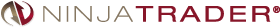The Leavitt indicators, as discussed by author Jay Leavitt in the December 2019 Letters to S&C column, are available for download at the following links for NinjaTrader 8 and for NinjaTrader 7:

You can review the indicator source code in NinjaTrader 8 by selecting the menu New → NinjaScript Editor → Indicators from within the control center window and selecting the LeavittProjection, LeavittConvolution, LeavittConvolutionSlope, and LeavittConvolutionAcceleration files. You can review the indicator’s source code in NinjaTrader 7 by selecting the menu Tools → Edit NinjaScript → Indicator from within the control center window and selecting the LeavittProjection, LeavittConvolution, LeavittConvolutionSlope, and LeavittConvolutionAcceleration files.

NinjaScript uses compiled DLLs that run native, not interpreted, to provide the highest performance possible.

A sample chart implementing the indicators is shown in Figure 6.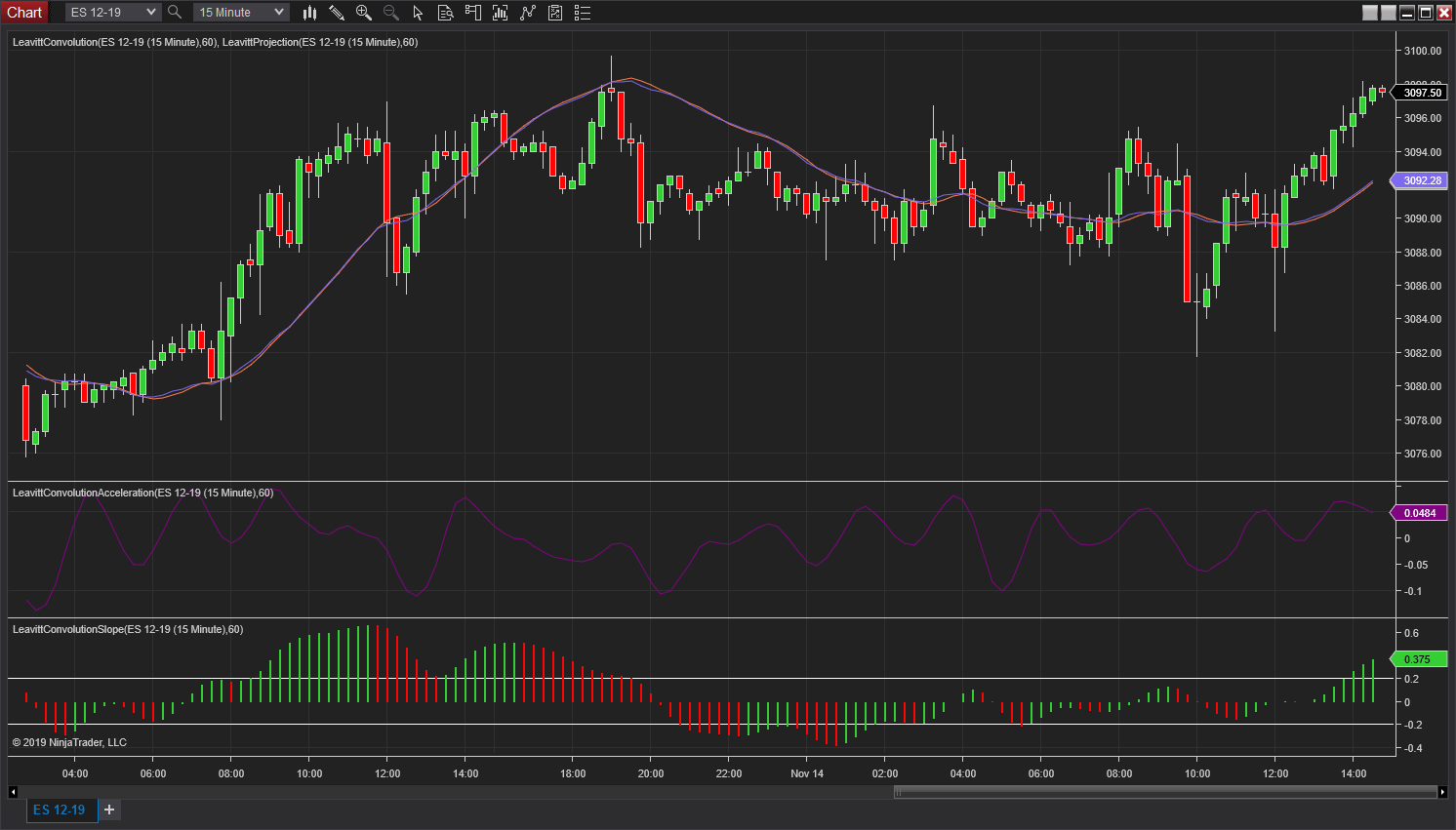FIGURE 6: NINJATRADER. The Leavitt indicators are displayed on a 15-minute ES 12–19 chart from November 13, 2019 to November 14, 2019.

—Raymond Deux & Chris Lauber

BACK TO LISTThe Leavitt projection and Leavitt convolution indicators can be easily implemented in NeuroShell Trader by combining a few of the NeuroShell Trader’s 800+ indicators. To implement the indicators, select “new indicator” from the insert menu and use the indicator wizard to set up the following indicators:

```LeavittProjection:	LinTimeReg PredValue(Close,10,1)
LeavittConv:	LinTimeReg PredValue(LeavittProjection,3,1)
LeavittConvSlope:	LinTimeReg Slope(LeavittProjection,3)
LeavittConvAcceleration:	Momentum(LeavittConvSlope,1)
LeavittConvSlope Indicator:	A>B(LeavittConvAcceleration,0)
```

Users of NeuroShell Trader can go to the Stocks & Commodities section of the NeuroShell Trader free technical support website to download a copy of this or any previous Traders’ Tips.

A sample chart is shown in Figure 7.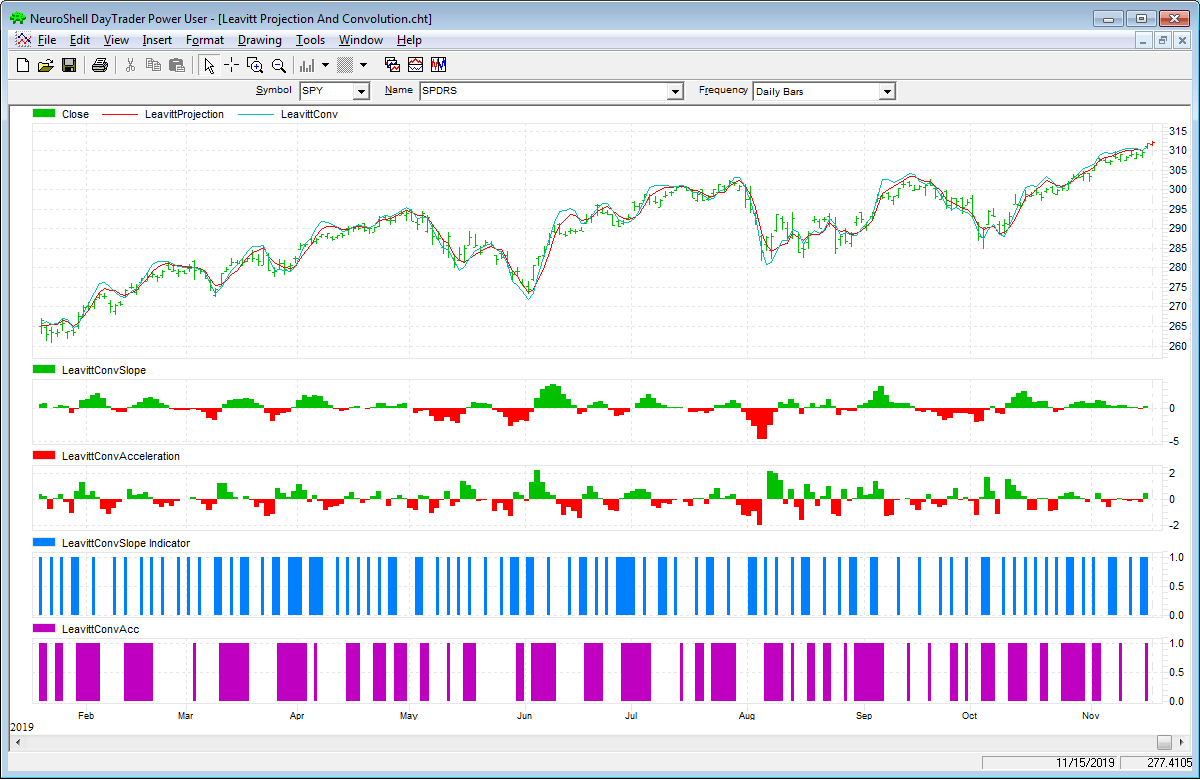FIGURE 7: NEUROSHELL TRADER. This NeuroShell Trader chart shows the Leavitt projection and convolution indicators.

—Marge Sherald, Ward Systems Group, Inc.
301 662-7950, sales@wardsystems.com
www.neuroshell.com

BACK TO LIST### AIQ: JANUARY 2020

The importable AIQ EDS file for Jay Leavitt’s article, “An Interplanetary Marriage,” can be obtained on request via email to info@TradersEdgeSystems.com. The code is also shown here:

```! AN INTERPLANETARY MARRIAGE
! Author: Jay A. Leavitt, TASC Jan 2020
! Coded by: Richard Denning, 11/17/2019

! CODE FOR LEAVITT PROJECTION:
! The Linear Regression Equation
!  The equation has the form Y= intercept + slope*X,
!  where Y is the dependent variable (that's the variable that goes on the Y axis),
!  X is the independent variable (i.e. it is plotted on the X axis),
!  in trading Y = end point of LR line as of today and X = number of time units

! INPUTS:
! how many days are we running this for?
constdays is floor(sqrt(100)).
days is HasDataFor(constdays).

! value (in this case the close)
varudf is [close].   !!!!!  y = varudf

! LINEAR REGRESSION CALCULATION (Least Means Squared method):
day is offsettodate(month(),day(),year())+1.
dayX2 is day * day.                         !!!  x = Day , dayX2 = x-squared

sx is Sum(day,days).                       !!!Sum of x
sx2 is Sum(dayX2,days).                !!!Sum of x*x
sy is Sum(varudf,days).                  !!!Sum of y
sy2 is Sum(varudf*varudf,days).
sxy is Sum( day * varudf,days).     !!!sum of x*y
d is ( days * sx2) - (sx * sx).

slope is ((days * sxy) - ( sx * sy)) / -d.  ! same result as internal Slope function
slopeInternal is slope2([close],days). ! display values on report to test calc

b is ((sx2 * sy) - (sx * sxy)) / d.
Intercept is slope + b.

!LINEAR REGRESSION LINE & NEXT DAY FORCAST:
!set days to 20 or more
LR is day*slope + intercept.
LRnext is (day+1)*slope + intercept. ! LEAVITT PROJECTION

ShowValues if 1.

!====================================================================================
! BE SURE TO PASTE THE FOLLOWING CODE INTO A SEPARATE EDS FILE:
! CODE FOR LEAVITT CONVOLUTION:
! The Linear Regression Equation
!  The equation has the form Y= intercept + slope*X,
!  where Y is the dependent variable (that's the variable that goes on the Y axis),
!  X is the independent variable (i.e. it is plotted on the X axis),
!  in trading Y = end point of LR line as of today and X = number of time units

! INPUTS:
! how many days are we running this for?
constdays is 100.
days is HasDataFor(constdays).

! value (in this case the close)
varudf is [close].   !!!!!  y = varudf

! LINEAR REGRESSION CALCULATION (Least Means Squared method):
day is offsettodate(month(),day(),year())+1.
dayX2 is day * day.                         !!!  x = Day , dayX2 = x-squared

sx is Sum(day,days).                       !!!Sum of x
sx2 is Sum(dayX2,days).                !!!Sum of x*x
sy is Sum(varudf,days).                  !!!Sum of y
sy2 is Sum(varudf*varudf,days).
sxy is Sum( day * varudf,days).     !!!sum of x*y
d is ( days * sx2) - (sx * sx).

slope is ((days * sxy) - ( sx * sy)) / -d.  ! same result as internal Slope function
slopeInternal is slope2([close],days). ! display values on report to test calc

b is ((sx2 * sy) - (sx * sxy)) / d.
Intercept is slope + b.

!LINEAR REGRESSION LINE & NEXT DAY FORCAST:
!set days to 20 or more
LR is day*slope + intercept.
LRnext is (day+1)*slope + intercept. !LEAVITT CONVOLUTION

ShowValues if 1.
```

I coded the indicators described by the author. Figure 8 shows the two indicators with a 100-bar length on a chart of the SPY. Please note that the code for each indicator must be pasted to separate EDS files. If both indicators are posted to a single EDS file, you will get a bunch of error messages.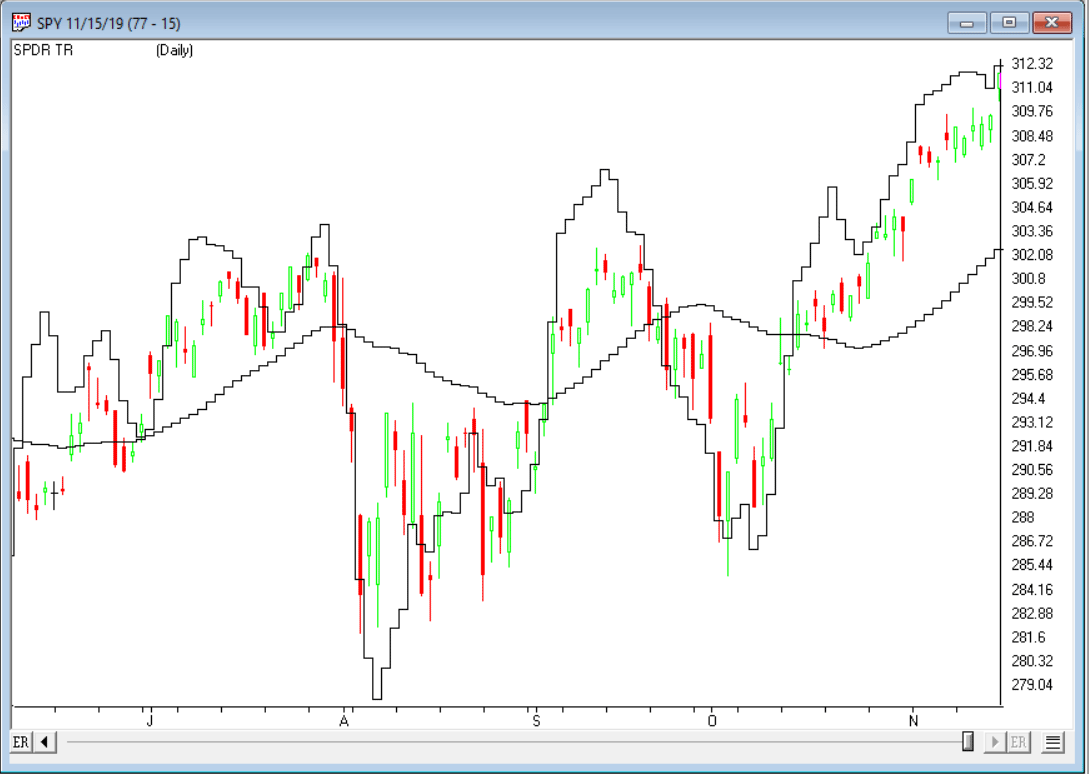FIGURE 8: AIQ. The two Leavitt indicators are shown on a chart of SPY.

—Richard Denning
for AIQ Systems

BACK TO LISTThe importable TradersStudio files for Jay Leavitt’s article, “An Interplanetary Marriage,” can be obtained on request via email to info@TradersEdgeSystems.com. The code is also shown here:

```' AN INTERPLANETARY MARRIAGE
' Author: Jay A. Leavitt, TASC Jan 2020
' Coded by: Richard Denning, 11/17/2019

'LEAVITT PROJECTION:
Sub LeavittProj_Ind(SLen)
Dim LeavittProj As BarArray
LeavittProj = LinearRegValue(C, SLen, -1)
plot1(LeavittProj)
End Sub

'------------------------------------------
'LEAVITT CONVOLUTION:
Sub LeavittConv_Ind(SLen)
Dim LeavittConv As BarArray
Dim SLenQ
SLenQ = Floor(Sqr(SLen))
LeavittConv = LinearRegValue(C, SLenQ, -1)
plot1(LeavittConv)
End Sub
```

I coded the indicators described by the author. Figure 9 shows the two indicators with a 100-bar length on a chart of the SPY.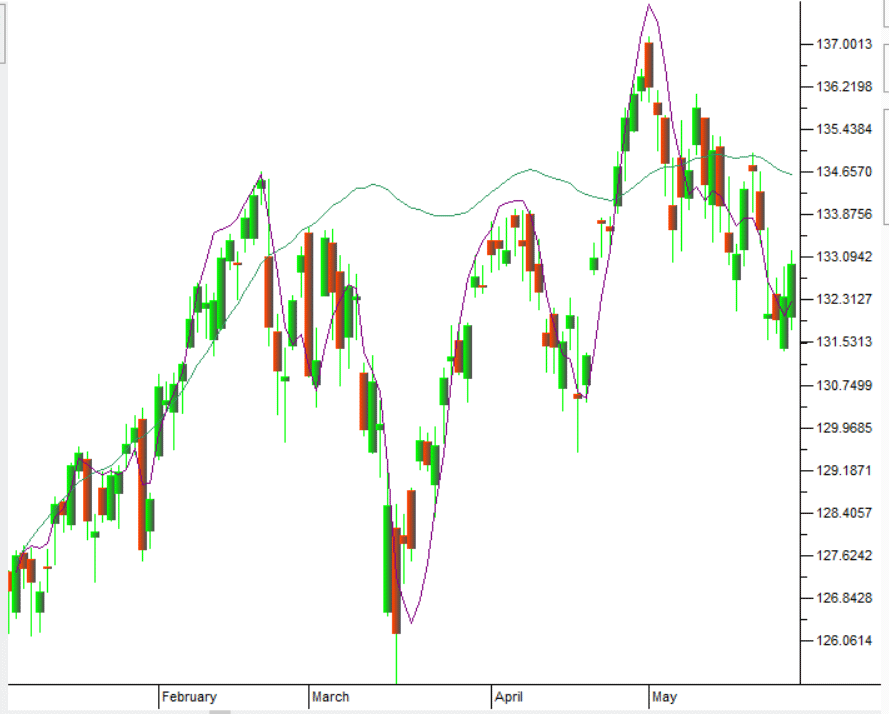FIGURE 9: TRADERSSTUDIO. The two Leavitt indicators are shown on a chart of SPY.

—Richard Denning